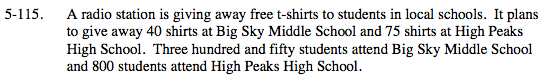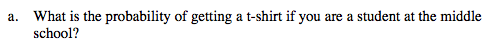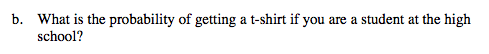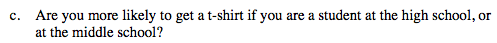### Home > MC2 > Chapter 5 > Lesson 5.3.3 > Problem5-115

5-115.Remember, probability can be expressed by this fraction:

$\frac{\text{number of shirts given at the school}}{\text{Total number of students at the school}}$

$\frac{40}{350}$See part (a).Convert each fraction to a percent. The school with the higher percentage means you are more likely to get a t-shirt.